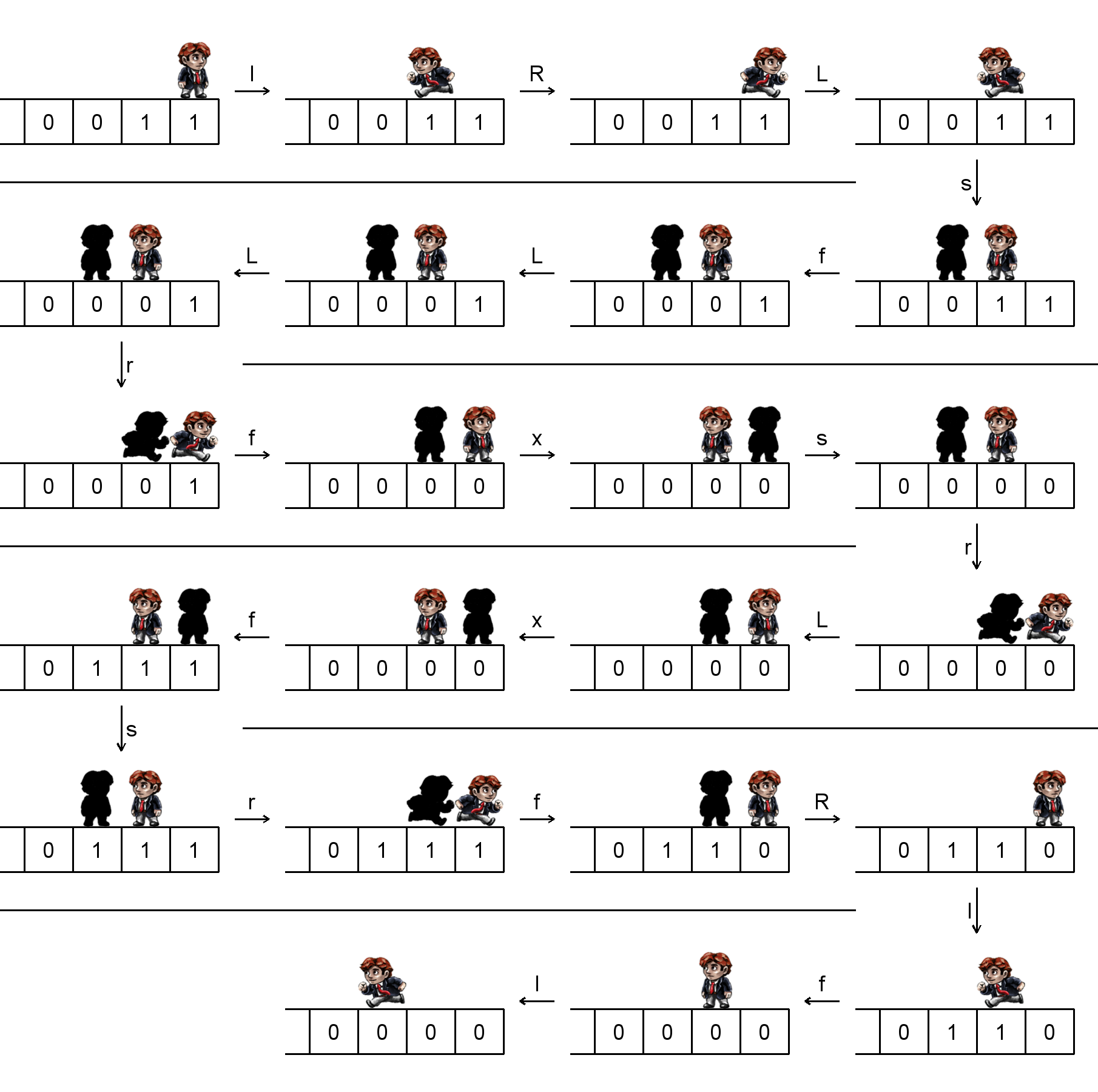시간 제한메모리 제한제출정답맞힌 사람정답 비율
2 초 512 MB76583.333%

## 문제

You are given a number n in its binary representation with infinite number of leading zeroes. You can walk over its bits and do some operations with them.

More precisely, you can make the following moves:

• “l”: walk one bit to the left. Your shadow, if it exists, does the same.
• “r”: walk one bit to the right. Your shadow, if it exists, does the same.
• “L”: if the bit you are standing at is 1 then walk one bit to the left, otherwise do nothing. Your shadow, if it exists and is standing at 1, also walks one bit to the left. Please note that you and your shadow behave independently of each other. You move if you are standing at 1, it moves if it is standing at 1.
• “R”: the same as the previous option, but you and your shadow move right instead of left.
• “x”: exchange positions with your shadow. If it does not exist, nothing happens.
• “f”: flip some bits (flipping means replacing 0 by 1 and vice versa). You flip two bits: the bit you are standing at and the bit to the left from you. Your shadow, if it exists, is not as strong as you, and flips only the bit it is standing at. Please note that during this move some bit may be flipped twice, thus remaining unchanged.

Initially you are at the rightmost (the least significant) bit, and 0 ≤ n < 210 holds. You are asked to write a program (that is, a sequence of moves) which satisfies the following:

• Neither you nor your shadow ever try to move to the right from the least significant bit (in other words, don’t try to leave the number).
• Your program consists of no more than 500 000 commands.
• The number at the end is n2.

Some technical details:

• Your position at the end may be arbitrary.
• Your shadow is allowed to exist in the end, and its position may be arbitrary.
• During the execution, the number represented by the bits may be arbitrarily large. The only constraint on it is that it must equal n2 in the end.
• Again, if you and your shadow flip the same bit simultaneously then it does not change.

## 입력

You will have no input.

## 출력

You should print a single string consisting of no more than 500 000 letters from the set “slrLRxf” representing your program.

## 예제 출력 1

lRLsfLLrfxsrLxfsrfRlfl


## 힌트

The sample output is incorrect. In fact, it is a program which, starting from the state where the two rightmost bits are x and y and the other bits are zeroes, comes to the state where all the bits are zeroes and the third bit (1-indexing) is the Sheffer stroke of x and y (which is ¬(x ∧ y)).

Below is an example of how it works for x = y = 1:## 채점 및 기타 정보

• 예제는 채점하지 않는다.